# 15 The Basics of Triangles

Click play on the following audio player to listen along as you read this section.You might be asking yourself right about now “Why do we learn about triangles in the trades?” Didn’t we finish with those way back in grade school?” Well believe it or not there are many situations where components or parts of systems in the trades have a triangle in their shape.

Knowing how to calculate the lengths of the sides of those triangles and even the angles involved might just come into play during your trades career.A good example of that would be a set of stairs. Take a look at the set of stairs to the left and note any triangle formed from those stairs.A carpenter making stairs will be required to know how many stairs will be in a stair case. He or she will also be required to know the height of the stairs as well as how the stairs are sloped. All in all the carpenter ends up using math, angles and triangles in the calculations.Another example would be when steam fitters are running pipe which changes direction. This change in direction is what is known as an offset. The change in direction might be 30 degrees, 60 degrees or 45 degrees. The properties of triangles are then used in order to calculate the dimensions of that offset.

Let’s start by identifying the parts of a triangle.

# Parts of a TriangleAs we all know a triangle has three sides and those sides have a relationship with each other depending on the angles between them.

The main characteristic of a triangle (other than the fact that it has three sides) is that the three angles within the triangle always add up to 180 degrees. No exceptions.

This means that angle “A” plus angle “B” plus angle “C” will add up to 180 degrees. If we know two of the angles it automatically means that we know the third.

For our purposes we will always be dealing with what is known as a right triangle. A right triangle is one in which one of the angles is 90 degrees. Take a look at the figure below to see what I mean.

In the figure to the left angle “B” is the right angle meaning that it’s 90 degrees.

This also means that angle “A” and angle “C” must add up to 90 degrees to make a total of 180 degrees.

The right angle is always identified by the box that it is enclosed in. Angles other than 90 degrees will be represented by a curved line.

The sides of the triangle also have names and those names all start with what is known as the identified angle. The identified angle will be the one which has the curved line attached to it. Take a look at the following triangle to see what I mean.

The right angle is always identified but this is not the angle we are referring to.

What we are referring to is angle “A”. It’s identified not only by the letter “A” but also by the curved line enclosing the angle.

This is our starting point.

I’ll give you the names of the three sides before letting you know which ones they are. See if you can figure out which is which just from the names.

1) Opposite

3) Hypotenuse

Which side do you think each of these refers to? Take a look at the following three diagrams to find out.

The opposite side is the side opposite the identified angle. Makes sense.

Following that same mode of thinking the adjacent side is the side directly adjacent to the identified angle.

Now if you are looking at the triangle and saying “wait a minute” there are two adjacent sides to the identified angle you are correct. The one we are referring to as the adjacent is the same side that has the 90 degree angle.

I’m going to guess that you figured out which side is the hypotenuse as there is only one option left. It doesn’t leave much room for debate.

The hypotenuse is always the longest of the three sides and is always directly opposite the 90 degree angle.

# Pythagorean TheoremThe next phase of our triangle education involves a guy with an interesting name. His name was Pythagoras and he lived a long time ago in Greece. He was a philosopher who is credited with a bunch of philosophical stuff as well as some math. One of the mathematical things he discovered was eventually named after him. The Pythagorean theorem.

His idea was that as long as you know two of the three sides in any right triangle you can figure out the third using a mathematical formula. Take a look at the following triangle to start. Always remember that the triangle must be a right triangle in order for the Pythagorean theorem  to work.

Note that the only angle that we know for sure is the right angle. It doesn’t actually matter what the other two angles are. Mathematically there is a definite relationship between the three sides………and here it is!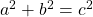That’s it. The most challenging part here is working with the equation and moving the numbers around so we can solve for any of the three sides. This goes back to an earlier chapter in the book where we learned how to work with formulas and transpose formulas. If you need a refresher on transposing please refer to the earlier chapter. There might also be a goofy video somewhere with me and that guy Chad in it.

We need to be able to solve for not only side “C” but also side “B” and side “A”.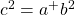From this we get…………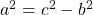And also…………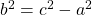That’s the three versions of the formula. The only problem is that everything is still squared. Finding an answer that is squared doesn’t work for us so what we end up doing is square rooting the answer to get our final answer.

Like so………..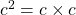And……….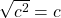Now going back to transposing equations we remember that whatever we do to one side we must do to the other. Therefore if we square root one side we must do that to the other side as well.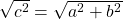And we get…..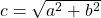This is the formula we’re required to work with and we can do the same thing for both side “A” and side “B”. Before we go any further take the formula and solve for both side “A” and side ‘B”. Once you have solved for both take a look down below to see if you were correct.Solving for side “A” or side “B” gets us the following: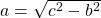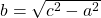What this means is that no matter what the angles are in a right triangle we can calculate the length of any of the sides as long as we have the lengths of the other two sides. Let’s go through an example.

Example

You have a right angle triangle. Side A is 12 and side B is 14. What is the length of side C?Step 1: Write down the formula and rearrange to solve for C.Step 2: Write down the variables.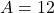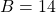Step 3: Plug the variables into the formula and work through it.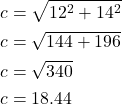Examples

What if we had to solve for side A? How would that look? Take a look at the following figure.

Step 1: Write down the formula you are going to use and rearrange the formula if necessary.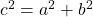Step 2: Write down the variables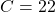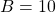Step 3: Plug the variables into the formula and work through it.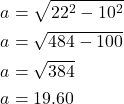Now try a couple questions for yourself. Make sure to check the video answers once you are done.

# Practice Questions